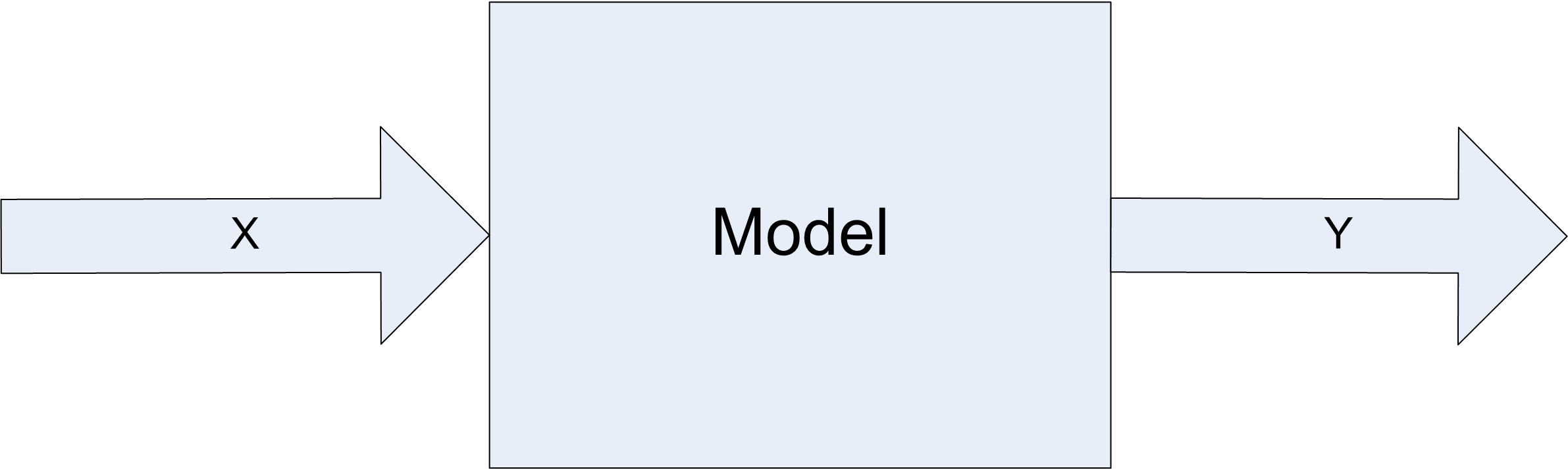# Chapter 3 Statistical Analysis for Finite Horizon Simulation Models

LEARNING OBJECTIVES

• To be able to recognize which type of planning horizon is applicable to a simulation study

• To be able to recognize the different types of statistical quantities used within and produced by the execution of simulation models

• To review statistical concepts and apply them to the analysis of finite horizon simulation studies

• To be able to analyze finite horizon simulations via the method of replications

• Introduce new Arena modeling constructs and how to use them in more complex modeling situations

• To be able to analyze and compare the results from two or more design configuration via simulation

As discussed in Chapter 1 and seen in Chapter 2 discrete event simulation is a very useful tool when randomness is an important aspect of the system environment and thus needs to be included in the simulation modeling. Because the inputs to the simulation are random, the outputs from the simulation are also random. This concept is illustrated in Figure 3.1. You can think of a simulation model as a function that maps inputs to outputs. This chapter presents the statistical analysis of the outputs from simulation models, specifically that of finite horizon simulations.

In addition, a number of issues that are related to the proper execution of simulation experiments are presented. For example, the simulation outputs are dependent upon the input random variables, input parameters, and the initial conditions of the model.Figure 3.1: Random input implies tandom output

Input parameters are related to the controllable and uncontrollable factors associated with the system. For a simulation model, all input parameters are controllable; however, in the system being modeled you typically have control over only a limited set of parameters. Thus, in simulation you have the unique ability to control the random inputs into your model. Chapter 4 will discuss how to take advantage of controlling the random inputs.

Input parameters can be further classified as decision variables. That is, those parameters of interest that you want to change in order to test model configurations for decision-making. The structure of the model itself may be considered a decision variable when you are trying to optimize the performance of the system. When you change the input parameters for the simulation model and then execute the simulation, you are simulating a different design alternative.

This chapter describes how to analyze the output from a single design alternative and how to analyze the results of multiple design alternatives. Before addressing these important concepts, we need to understand how the time horizon of a simulation study is determined and how it might affect the statistical methods that will be required during the analysis.